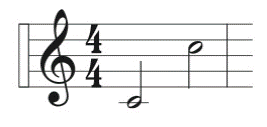Chapter 13.3, Problem 92E### Algebra and Trigonometry (MindTap ...

4th Edition
James Stewart + 2 others
ISBN: 9781305071742

#### Solutions

Chapter
Section### Algebra and Trigonometry (MindTap ...

4th Edition
James Stewart + 2 others
ISBN: 9781305071742
Textbook Problem

# Musical Frequencies The frequencies of musical notes (measured in cycles per second) form a geometric sequence. Middle C has a frequency of 256 , and the C that is an octave higher has a frequency of 512 . Find the frequency of C two octaves below middle C .To determine

The frequency of C two octaves below middle C.

Explanation

Given:

1) The frequencies of musical notes (measured in cycles per second) form a geometric sequence.

2) Middle C has a frequency of 256.

3) The C that is an octave higher has a frequency of 512.

Approach:

1 Octave = 2 Notes

Calculation:

Middle C has a frequency of 256 and the C that is an octave higher has a frequency of 512.

So, for each octave, the frequencies increase by a factor of 2 as shown in Figure (1).

### Still sussing out bartleby?

Check out a sample textbook solution.

See a sample solution

#### The Solution to Your Study Problems

Bartleby provides explanations to thousands of textbook problems written by our experts, many with advanced degrees!

Get Started